# Complex integration, method of

method of contour integration

One of the universal methods in the study and applications of zeta-functions,-functions (cf. Zeta-function;-function) and, more generally, functions defined by Dirichlet series.

The method of complex integration was first introduced by B. Riemann  in 1876 into number theory in connection with the study of the properties of the zeta-function. The well-known modern applications of the method of complex integration, which use the Cauchy theorem on residues, the Phragmén–Lindelöf theorem on Dirichlet series, the saddle point method, etc., are extremely varied in their form and content. The method of complex integration is used for the analytic continuation and the derivation of functional equations of Dirichlet functions; for the derivation of approximate functional equations of these functions; for estimating the number of their non-trivial zeros and the density of the distribution of these zeros in some part of the critical strip; and for obtaining asymptotic formulas and estimates of various kinds of the most important arithmetic functions. A classical example of the method of complex integration is illustrated by the proof of the analytic continuation and the derivation of the functional equation of the Riemann zeta-function (see , ). For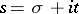,, one has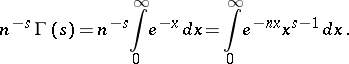after summation one finds that the function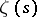, originally defined by the series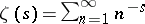for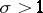, is also expressed by the formula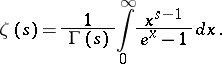(1)

Consider the integral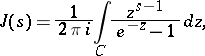taken along the (infinite) contour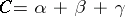, where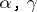pass along the lower and upper edges of the cut along the negative real axis of the-plane andis a circle of radius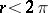passing round the origin. The integral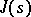converges for all, the convergence being uniform in any disc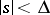, since the integrand is less than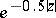onandfor all. By Cauchy's theorem it does not depend on, hence is an entire function of. Assuming that on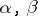and, respectively,,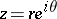,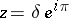, and, it is easy to writeas a sum of integrals with respect to real variables: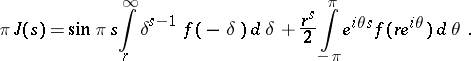In the disc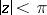one has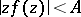. Therefore the second term on the right-hand side of the equation is less than, which for any fixedwithconverges to zero as. Hence, by formula (1),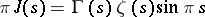and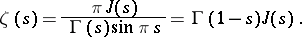(2)

This formula, proved under the hypothesis that, provides a continuation ofto the whole plane. It is clear from this thatis a single-valued analytic function on the whole-plane, having as its only singularity a simple pole at the point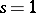with residue.

To deduce the functional equation ofit is assumed that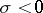and thatis an integer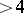. Let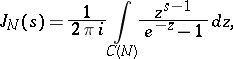whereis a contour that differs from the previous contour by joiningandby a circular arc of radiuswith centre at the origin. The integral along the outer arc of the contour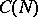can be estimated in the form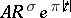, which forconverges to zero as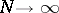. Henceas. On the other hand, by the residue theorem,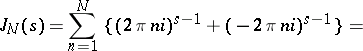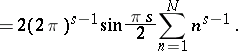Therefore, when,This equation, in combination with formula (2), gives the relation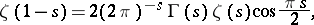which, according to the theory of analytic continuation, holds throughout the whole-plane. It is called the functional equation of the Riemann zeta-function.

The method of complex integration plays an important role in obtaining the approximate functional equations which lie at the basis of modern estimates of Dirichlet functions (see , ).

The method of complex integration is fundamental in the study of the distribution of zeros of the functions,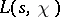, etc. Until recently it has been applied in the form of the well-known Littlewood theorem on the number of zeros in a rectangle of a functionthat is regular for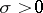, the Bäcklund theorem about, and also to theorems about convexity of mean values of analytic functions (see ). In 1969, H. Montgomery  found a new, direct, more powerful way of applying the method of complex integration to these ends.

The method of complex integration in its applications to number theory naturally arises in connection with summation formulas for the coefficients of Dirichlet series (see , ).